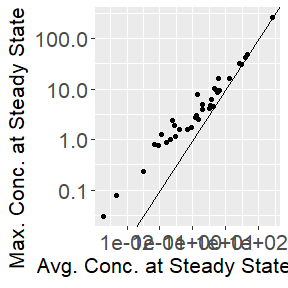# Pearce et al. (2017): Updated v79i04.R Examples

#### 2023-02-20

from “httk: R Package for High-Throughput Toxicokinetics”

Robert G Pearce, R Woodrow Setzer, Cory L Strope, Nisha S Sipes, and John F Wambaugh

Journal of Statistical Software 2017 Jul 17; 79(4): 1-26.

https://doi.org/10.18637%2Fjss.v079.i04

## Abstract

Thousands of chemicals have been profiled by high-throughput screening programs such as ToxCast and Tox21; these chemicals are tested in part because most of them have limited or no data on hazard, exposure, or toxicokinetics. Toxicokinetic models aid in predicting tissue concentrations resulting from chemical exposure, and a “reverse dosimetry” approach can be used to predict exposure doses sufficient to cause tissue concentrations that have been identified as bioactive by high-throughput screening. We have created four toxicokinetic models within the R software package httk. These models are designed to be parameterized using high-throughput in vitro data (plasma protein binding and hepatic clearance), as well as structure-derived physicochemical properties and species-specific physiological data. The package contains tools for Monte Carlo sampling and reverse dosimetry along with functions for the analysis of concentration vs. time simulations. The package can currently use human in vitro data to make predictions for 553 chemicals in humans, rats, mice, dogs, and rabbits, including 94 pharmaceuticals and 415 ToxCast chemicals. For 67 of these chemicals, the package includes rat-specific in vitro data. This package is structured to be augmented with additional chemical data as they become available. Package httk enables the inclusion of toxicokinetics in the statistical analysis of chemicals undergoing high-throughput screening.

This vignette was created from the R replication code v79i04.R for the 2017 paper that introduced httk.

## HTTK Version

The R replication code v79i04.R was created with httk v1.7. It was updated to a vignette using httk v2.2.2 in 2022. Although we attempt to maintain backward compatibility, if you encounter issues with the latest release of httk and cannot easily address the changes, historical versions of httk are available from: https://cran.r-project.org/src/contrib/Archive/httk/

## Prepare R to run the vignette

R package knitr generates html and PDF documents from this RMarkdown file, Each bit of code that follows is known as a “chunk”. We start by telling knitr how we want our chunks to look.

DTXSID=ids$dtxsid, CAS=this.cas) this.row <- cbind(this.row, as.data.frame(calc_analytic_css(chem.cas = this.cas, model = "pbtk", output.units = "uM", suppress.messages=TRUE))) this.row <- cbind(this.row, as.data.frame(get_wetmore_css(chem.cas = this.cas, which.quantile = 0.50, output.units = "uM", suppress.messages=TRUE))) this.row <- cbind(this.row, as.data.frame(calc_mc_css(chem.cas = this.cas, which.quantile = 0.50, output.units = "uM", samples = NSAMP, suppress.messages=TRUE))) css.table <- rbind(css.table, this.row) } colnames(css.table) <- c("Compound","DTXSID","CAS", "PBTK", "Wetmore", "MC") Now display the table and plot two columns against each other: knitr::kable(css.table, caption = "Literature and HTTK Plasma Css", floating.environment="sidewaystable", row.names=FALSE, digits=3) Literature and HTTK Plasma Css Compound DTXSID CAS PBTK Wetmore MC 2,4-d DTXSID0020442 94-75-7 43.990 43.320 50.670 Buprofezin DTXSID8034401 69327-76-0 42.660 9.163 123.300 Dicrotophos DTXSID9023914 141-66-2 1.949 0.489 3.554 Flufenacet DTXSID2032552 142459-58-3 0.303 0.179 0.519 Isoxaben DTXSID8024159 82558-50-7 1.493 24.460 3.655 Oxytetracycline dihydrate DTXSID4023412 6153-64-6 1.967 1.938 4.224 Rotenone DTXSID6021248 83-79-4 3.460 1.833 10.950 Triflusulfuron-methyl DTXSID2032502 126535-15-7 0.094 0.106 0.177 “4-(1,1,3,3-tetramethylbutyl)phenol” DTXSID9022360 140-66-9 4.309 0.131 1.876 3-phenoxybenzoic acid DTXSID1038321 3739-38-6 253.700 357.600 389.600 Sulfasalazine DTXSID0021256 599-79-1 25.570 23.430 51.710 Ethoxyquin DTXSID9020582 91-53-2 5.409 0.624 14.560 litvshttkcss <- ggplot(css.table, aes(Wetmore, MC)) + geom_point() + geom_abline() + scale_x_log10() + scale_y_log10() + xlab(bquote("Literature"~C[ss]~"("*mu*"M)")) + ylab(bquote("HTTK"~C[ss]~"("*mu*"M)")) + theme(axis.text = element_text(size = 16), axis.title = element_text(size = 16)) print(litvshttkcss)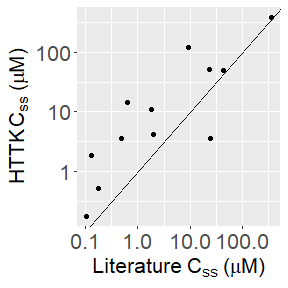## Plotting example To see how $$C_{ss}$$ resulting from discrete dosing deviates from the average steady state concentration, we can make a plot with ggplot2 that includes a horizontal line through the $$y$$-axis at the predicted $$C_{ss}$$ for oral infusion dosing (Figure 2 in Pearce et al., 2017). out <- solve_pbtk(chem.name = "Bisphenol A", days = 50, doses.per.day = 3, daily.dose=1, output.units = "uM") #> Warning in get_clint(dtxsid = dtxsid, chem.name = chem.name, chem.cas = #> chem.cas, : Clint is provided as a distribution. #> Warning in apply_clint_adjustment(Clint.point, Fu_hep = Fu_hep, #> suppress.messages = suppress.messages): Clint adjusted for in vitro #> partitioning (Kilford, 2008), see calc_hep_fu. #> Warning in get_fup(dtxsid = dtxsid, chem.name = chem.name, chem.cas = chem.cas, #> : Fraction unbound is provided as a distribution. #> Warning in apply_fup_adjustment(fup.point, fup.correction = fup.adjustment, : #> Fup adjusted for in vivo lipid partitioning (Pearce, 2017), see #> calc_fup_correction. #> Warning in available_rblood2plasma(chem.cas = chem.cas, species = species, : #> Human in vivo measured Rblood2plasma used. #> None of the monitored components undergo unit conversions (i.e. conversion factor of 1). #> #> AUC is area under the plasma concentration curve in uM*days units with Rblood2plasma = 0.795. #> The model outputs are provided in the following units: #> umol: Agutlumen, Atubules, Ametabolized #> uM: Cgut, Cliver, Cven, Clung, Cart, Crest, Ckidney, Cplasma #> uM*days: AUC plot.data <- as.data.frame(out) css <- calc_analytic_css(chem.name = "Bisphenol A", output.units = "uM") #> Warning in get_clint(dtxsid = dtxsid, chem.name = chem.name, chem.cas = #> chem.cas, : Clint is provided as a distribution. #> Warning in apply_clint_adjustment(Clint.point, Fu_hep = Fu_hep, #> suppress.messages = suppress.messages): Clint adjusted for in vitro #> partitioning (Kilford, 2008), see calc_hep_fu. #> Warning in get_fup(dtxsid = dtxsid, chem.name = chem.name, chem.cas = chem.cas, #> : Fraction unbound is provided as a distribution. #> Warning in apply_fup_adjustment(fup.point, fup.correction = fup.adjustment, : #> Fup adjusted for in vivo lipid partitioning (Pearce, 2017), see #> calc_fup_correction. #> Warning in available_rblood2plasma(chem.cas = chem.cas, species = species, : #> Human in vivo measured Rblood2plasma used. #> Plasma concentration returned in uM units. c.vs.t <- ggplot(plot.data, aes(time, Cplasma)) + geom_line() + geom_hline(yintercept = css) + ylab(bquote("Plasma Concentration ("*mu*"M)")) + xlab("Day") + theme(axis.text = element_text(size = 16), axis.title = element_text(size = 16), plot.title = element_text(size = 17, hjust=0.5)) + ggtitle("Bisphenol A") print(c.vs.t)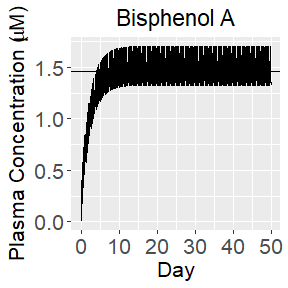### Suppressing Messages Note that since in vitro-in vivo extrapolation (IVIVE) is built upon many, many assumptions, *httk** attempts to give many warning messages by default. These messages do not usually mean something is wrong, but are rather intended to make the user aware of the assumptions involved. However, they quickly grow annoying and can be turned off with the “suppress.messages=TRUE” argument. Proceed with caution… out <- solve_pbtk(chem.name = "Bisphenol A", days = 50, doses.per.day = 3, daily.dose=1, output.units = "uM", suppress.messages = TRUE) css <- calc_analytic_css(chem.name = "Bisphenol A", output.units = "uM", suppress.messages = TRUE) ## TK Study Summary Statistics “calc_tk_stats” allows summary TK statistics to be calculated for a specific study design (including dose regiment, duration, and species). The following code calculates the peak plasma concentration for all chemicals in httk simulated for 10 days at 1 mg/kg BW/day with 3 doses per day. Note that the function “calc_stats” was renamed “calc_tk_stats” in a later release of httk. all.peak.stats <- calc_stats(days = 10, doses.per.day = 3, stats = "peak") The same function can be used for a single chemical. For example, the AUC, peak, and mean for a single 1 mg dose of Triclosan over 10 day: triclosan.stats <- calc_stats(days = 10, chem.name = "triclosan") #> Warning in calc_stats(days = 10, chem.name = "triclosan"): Function #> "calc_stats" has been renamed to "calc_tkstats". #> Human plasma concentrations returned in uM units. #> AUC is area under plasma concentration curve in uM * days units with Rblood2plasma = 0.6436 . ## Steady-state Plasma Concentration Below are examples of two functions,comparing the medians of the Wetmore data in humans for 1 mg/kg BW/day of Bisphenol A with the “calc_mc_css” simulation with probability distributions containing a third of the standard deviation, half the limit of detection for $$f_{up}$$, and the same number of samples of the parameters used in Wetmore et al. (2012). We use make use of the default Monte Carlo sampler by setting argument “httkpop=FALSE” to turn off httk-pop (Ring et al., 2017) and “invitrouv=FALSE” to turn off uncertainty/variability for the in vitro parameters (Wambaugh et al., 2019). Note that the function “get_wetmore_css” was renamed “get_lit_css” in a later release of httk. get_wetmore_css(chem.cas = "80-05-7", daily.dose = 1, which.quantile = 0.5, output.units = "uM") #> Warning in get_wetmore_css(chem.cas = "80-05-7", daily.dose = 1, which.quantile #> = 0.5, : Function "get_wetmore_css" has been renamed to "get_lit_cheminfo". #> Human plasma concentrations returned in uM units. #> Retrieving Css from literature based on 1 uM intrinsic clearance data for the 0.5 quantile in Human. #>  0.8569 set.seed(654321) calc_mc_css(chem.cas = "80-05-7", daily.dose = 1, which.quantile = 0.5, censored.params = list( Funbound.plasma = list(cv = 0.1, lod = 0.005)), vary.params = list( BW = 0.15, Vliverc = 0.15, Qgfrc = 0.15, Qtotal.liverc = 0.15, million.cells.per.gliver = 0.15, Clint = 0.15), output.units = "uM", samples = NSAMP, httkpop=FALSE, invitrouv=FALSE, suppress.messages = TRUE) #> 50% #> 1.752 ### Using more sophisticated Monte Carlo httk uses Monte Carlo to simulate population variability and propagate parameter uncertainty. The algorithm for population variability, “httk-pop” (Ring et al., 2017) simulates population variability in TK by predicting distributions of physiological parameters based on distributions of demographic and biometric quantities from the National Health and Nutrition Examination Survey (NHANES), conducted by the U.S. Centers for Disease Control and Prevention (CDC). MEthods for propagating uncertainty in in vitro measurements were described by Wambaugh et al. (2019). Both httk-pop and in vitro uncertainty/variability simulation, are on by default: set.seed(654321) calc_mc_css(chem.cas = "80-05-7", daily.dose = 1, which.quantile = 0.5, output.units = "uM", samples = NSAMP, suppress.messages = TRUE) #> 50% #> 3.446 ### Controlling the random number generator Any of the Monte Carlo functions in httk, often indicated by the inclusion of “mc” in the function name, make use of random draws from distributions to simulate uncertainty and variability. The random number generator in any computer is actually a pseudo-random number generator that creates a sequence of nearly uncorrelated numbers, but that sequence can be recreated at any time if we set a random number generator “seed”. In R we control the seed with the function “set.seed”, which allows us to get the same Monte Carlo output again and again (and in many cases on different computers). The seed can take any value, and if you use the same seed followed by the same functions called in the same order with the same arguments, you will get the same answer again and again: # Random number generator seed 1: set.seed(654321) calc_mc_css(chem.cas = "80-05-7", daily.dose = 1, which.quantile = 0.5, output.units = "uM", samples = NSAMP, suppress.messages = TRUE) #> 50% #> 3.446 # Continuing to draw random numbers without resetting the seed: calc_mc_css(chem.cas = "80-05-7", daily.dose = 1, which.quantile = 0.5, output.units = "uM", samples = NSAMP, suppress.messages = TRUE) #> 50% #> 2.326 # Random number generator seed 2: set.seed(123456) calc_mc_css(chem.cas = "80-05-7", daily.dose = 1, which.quantile = 0.5, output.units = "uM", samples = NSAMP, suppress.messages = TRUE) #> 50% #> 3.013 # Continuing to draw random numbers without resetting the seed: calc_mc_css(chem.cas = "80-05-7", daily.dose = 1, which.quantile = 0.5, output.units = "uM", samples = NSAMP, suppress.messages = TRUE) #> 50% #> 2.972 # Random number generator seed 2 gives the same answer as it did above: set.seed(123456) calc_mc_css(chem.cas = "80-05-7", daily.dose = 1, which.quantile = 0.5, output.units = "uM", samples = NSAMP, suppress.messages = TRUE) #> 50% #> 3.013 # Random number generator seed 1 gives the same answer as it did above: set.seed(654321) calc_mc_css(chem.cas = "80-05-7", daily.dose = 1, which.quantile = 0.5, output.units = "uM", samples = NSAMP, suppress.messages = TRUE) #> 50% #> 3.446 ## IVIVE with HTTK Simple “reverse dosimetry” in vitro-in vivo extrapolation (IVIVE) determines an administered equivalent dose (AED) by taking an in vitro concentration $$C_{\text{in vitro}}$$ (50 uM in the example below) and dividing it by the steady-state plasma concentration $$C_{ss}$$ produced by a dose rate of 1 mg/kg bw/day: $AED = \frac{C_{\text{in vitro}}}{C_{ss}}$ We can also calculate the AED using literature values or sampling with httk: get_wetmore_oral_equiv(50, chem.cas = "80-05-7") #> Warning in get_wetmore_oral_equiv(50, chem.cas = "80-05-7"): Function #> "get_wetmore_oral_equiv" has been renamed to "get_lit_oral_equiv". #> Retrieving Css from literature based on 10 uM intrinsic clearance data for the 0.95 quantile in Human. #> Human uM concentration converted to mg/kg bw/day dose. #>  37.06 set.seed(654321) calc_mc_oral_equiv(50, chem.cas = "80-05-7", samples = NSAMP) #> uM concentration converted to mgpkgpday dose for 0.95 quantile. #> 95% #> 2.463 ### Monte Carlo Simulation of $$C_{ss}$$ We can perform a Monte Carlo simulation on Zoxamide with the model pbtk with the same limit of detection and coefficients of variation (cv). Note, the function “monte_carlo” was replaced with a similar function “calc_mc_tk” for simulation concentration timecourses, while arguments were added to “calc_mc_css” that allowed the functionality seen in the 2017 paper. Separately, a new function “monte_carlo” was created to complement “httkpop_mc” and “invitro_mc” as part of “create_mc_samples”. “monte_carlo” varies parameters according to a normal distribution with given mean and coefficient of variation or according to a censored distribution with an additional limit of detection parameter. Note that we again turn off httkpop and invitrouv to replicate the 2017 version of Monte Carlo. First we create a list of parameters and then assign a coefficient of variation to each parameter we wish to vary: vary.params <- NULL params <- parameterize_pbtk(chem.name = "Zoxamide") #> Warning in apply_clint_adjustment(Clint.point, Fu_hep = Fu_hep, #> suppress.messages = suppress.messages): Clint adjusted for in vitro #> partitioning (Kilford, 2008), see calc_hep_fu. #> Warning in calc_ma(chem.cas = chem.cas, chem.name = chem.name, dtxsid = dtxsid, #> : Membrane affintity (MA) predicted with method of Yun and Edginton (2013), see #> calc_ma. #> Warning in apply_fup_adjustment(fup.point, fup.correction = fup.adjustment, : #> Fup adjusted for in vivo lipid partitioning (Pearce, 2017), see #> calc_fup_correction. #> Warning in available_rblood2plasma(chem.cas = chem.cas, species = species, : #> Human in vivo measured Rblood2plasma used. for (this.param in names(subset(params, names(params) != "Funbound.plasma"))) # Only want to vary numerical parameters: if (is.numeric(params[[this.param]])) vary.params[this.param] <- 0.2 We can draw $$f_{up}$$ from a censored distribution with a limit of dextion (LOD) of 0.01: censored.params <- list(Funbound.plasma = list(cv = 0.2, lod = 0.01)) ### Solve the full TK time-course set.seed(1) out <- calc_mc_tk(parameters=params, vary.params = vary.params, censored.params = censored.params, return.samples = TRUE, model = "pbtk", suppress.messages = TRUE, httkpop = FALSE, invitrouv = FALSE, samples = NSAMP, solvemodel.arg.list = list(times = seq(0,0.5,0.025)) ) Make a table of concentration vs. time with confidence intervals: zoxtable <- cbind(out[["means"]][,"time"], out[["means"]][,"Cplasma"], out[["means"]][,"Cplasma"] - 1.96*out[["sds"]][,"Cplasma"], out[["means"]][,"Cplasma"] + 1.96*out[["sds"]][,"Cplasma"]) colnames(zoxtable) <- c("time","Cplasma","lcl","ucl") knitr::kable(zoxtable, caption = "Zoxamide plasma concentration vs. time", floating.environment="sidewaystable") Zoxamide plasma concentration vs. time time Cplasma lcl ucl 0.000 0.000 0.000000 0.000000 0.025 2.956 2.662392 3.249608 0.050 2.448 2.285163 2.610837 0.075 2.151 1.829364 2.472636 0.100 2.034 1.584180 2.483820 0.125 1.991 1.473168 2.508832 0.150 1.975 1.426396 2.523604 0.175 1.969 1.407460 2.530540 0.200 1.967 1.400168 2.533832 0.225 1.966 1.397208 2.534792 0.250 1.966 1.396228 2.535772 0.275 1.965 1.395228 2.534772 0.300 1.965 1.395032 2.534968 0.325 1.965 1.395032 2.534968 0.350 1.965 1.395032 2.534968 0.375 1.965 1.395032 2.534968 0.400 1.965 1.395032 2.534968 0.425 1.964 1.394228 2.533772 0.450 1.964 1.394228 2.533772 0.475 1.964 1.394228 2.533772 0.500 1.964 1.394228 2.533772 Plot Monte Carlo analysis of concentration vs. time: zoxamide1 <- ggplot(as.data.frame(zoxtable), aes(x=time,y=Cplasma)) + geom_line(color = "dark blue",size=2) + geom_ribbon(aes(ymin=lcl, ymax=ucl), alpha = 0.3, fill = "light blue", color="black", linetype="dotted")+ ylab("Plasma concentration") + xlab("Time (days)") + theme(axis.text = element_text(size = 16), axis.title = element_text(size = 16)) print(zoxamide1)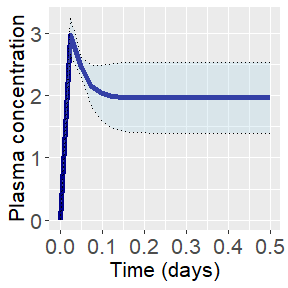### Examine $$C_{ss}$$ out <- calc_mc_css(parameters=params, vary.params = vary.params, censored.params = censored.params, return.samples = TRUE, model = "pbtk", suppress.messages = TRUE, httkpop = FALSE, samples = NSAMP, invitrouv = FALSE) zoxamide2 <- ggplot(as.data.frame(out), aes(out)) + geom_histogram(fill = "blue", binwidth = 1/6) + scale_x_log10() + ylab("Number of Samples") + xlab(bquote(C[ss]~"("*mu*"M)")) + theme(axis.text = element_text(size = 16), axis.title = element_text(size = 16)) print(zoxamide2)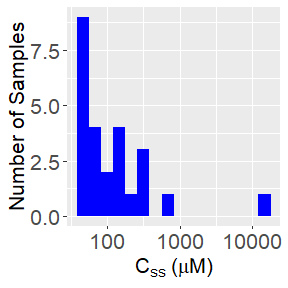## Adding a tissue The httk package contains a data.frame called tissue.data that describes the composition of various tissues in terms of the Schmitt (2008) mechanistic model for deriving tissue:plasma partition coefficients. Once described, these tissues are available for use as compartments in new models or to be lumped into the rest of body in existing models (using function “lump_tissues”). We can add a new tissue (for example, mammary) to the tissue data by replicating the data from another tissue and adjusting as appropriate. new.tissue <- subset(tissue.data,Tissue == "adipose" & Species =="Human") new.tissue[, "Tissue"] <- "mammary" new.tissue[new.tissue$variable=="Vol (L/kg)","value"] <-
320/1000/60 # Itsukage et al. (2017) PMID: 29308107
new.tissue[new.tissue$variable %in% c('Flow (mL/min/kg^(3/4))'),'value'] <- # We'll arbitrarily set flow to a tenth of total adipose: new.tissue[new.tissue$variable %in% c('Flow (mL/min/kg^(3/4))'),'value']/10
tissue.data <- rbind(tissue.data, new.tissue)
knitr::kable(subset(tissue.data,Tissue=="mammary"),
caption = "Approximate Mamary Tissue Parameters",
floating.environment="sidewaystable",
row.names=FALSE,
digits=3)
Approximate Mamary Tissue Parameters
Tissue Species Reference variable value
mammary Human Schmitt 2008 Fcell 0.860
mammary Human Schmitt 2008 Fint 0.140
mammary Human Schmitt 2008 FWc 0.020
mammary Human Schmitt 2008 FLc 0.934
mammary Human Schmitt 2008 FPc 0.046
mammary Human Schmitt 2008 Fn_Lc 0.936
mammary Human Schmitt 2008 Fn_PLc 0.056
mammary Human Schmitt 2008 Fa_PLc 0.008
mammary Human Schmitt 2008 pH 7.100
mammary Human EPA, Physiological Parameters Database for PBPK Modeling Density (g/cm^3) 0.916
mammary Human ILSI-RSI 1994 Vol (L/kg) 0.005
mammary Human Davies and Morris 1993 Flow (mL/min/kg^(3/4)) 1.074

If we thought this tissue had been included in the rest of body total previously we could consider removing it from those volumes and flows, but we won’t do that here (Note eval=FALSE):

# If we thought this tissue was included in the rest of the bod
tissue.data[tissue.data$Tissue == "rest", 'value'] <- tissue.data[tissue.data$Tissue == "rest", 'value'] -
new.tissue[new.tissue$variable %in% c( 'Vol (L/kg)', 'Flow (mL/min/kg^(3/4))'), 'value'] To generate the parameters for a model with kidneys, thyroid, brain, breast, liver compartment combining the liver and gut, and a rest of body compartment: compartments <- list(liver = c("liver", "gut"), kidney = "kidney", breast = "mammary", brain = "brain", thyroid = "thyroid") tissues.to.include <- unique(tissue.data$Tissue)
tissues.to.include <- tissues.to.include[!(tissues.to.include=="placenta")]
Kp <- predict_partitioning_schmitt(
chem.name = "Nicotine",
tissues = tissues.to.include,
suppress.messages = TRUE)
lumped.params <- lump_tissues(Kp, tissuelist=compartments)
knitr::kable(as.data.frame(lumped.params),
caption = "PCs, Volumes, and Flows for Lumped Tissues",
floating.environment="sidewaystable",
row.names=FALSE,
digits=3)
PCs, Volumes, and Flows for Lumped Tissues
Krbc2pu Kliver2pu Kkidney2pu Kbreast2pu Kbrain2pu Kthyroid2pu Krest2pu Vliverc Vkidneyc Vbreastc Vbrainc Vthyroidc Vrestc Qtotal.liverf Qkidneyf Qbreastf Qbrainf Qthyroidf Qrestf Qgutf
0.802 4.745 5.146 11.58 1.45 1.446 1.914 0.04 0.004 0.005 0.019 0 0.764 0.464 0.221 0.005 0.125 0.016 0.376 0.205

The httk package contains a data.frame called physiology.data that describes the species-specific aspects of physiology necessary to parameterize a PBTK model. We can add a new species (for example, wolverines) to the physiology.data by replicating the data from another species and adjusting as appropriate.

new.species <- physiology.data[,"Rabbit"]
names(new.species) <- physiology.data[,"Parameter"]
rabbit.BW <- new.species["Average BW"]
new.species["Average BW"] <- 31.2 # Rausch and Pearson (1972) https://doi.org/10.2307/3799057
new.species["Average Body Temperature"] <- 38.5 # Thiel et al. (2019) https://doi.org/10.1186/s12983-019-0319-8

physiology.data <- cbind(physiology.data, new.species)
colnames(physiology.data)[length(colnames(physiology.data))] <- "Wolverine"

knitr::kable(physiology.data[,c("Parameter","Units","Wolverine")],
caption = "Approximate Wolverine Physiological Parameters",
floating.environment="sidewaystable",
row.names=FALSE,
digits=3)
Approximate Wolverine Physiological Parameters
Parameter Units Wolverine
Total Body Water ml/kg 716.000
Plasma Volume ml/kg 44.000
Cardiac Output ml/min/kg^(3/4) 212.000
Average BW kg 31.200
Total Plasma Protein g/ml 0.057
Plasma albumin g/ml 0.039
Plasma a-1-AGP g/ml 0.001
Hematocrit fraction 0.360
Urine ml/min/kg^(3/4) 0.042
Bile ml/min/kg^(3/4) 0.083
GFR ml/min/kg^(3/4) 3.120
Average Body Temperature C 38.500
Plasma Effective Neutral Lipid Volume Fraction unitless 0.002
Plasma Protein Volume Fraction unitless 0.057
Pulmonary Ventilation Rate l/h/kg^(3/4) 24.750
Alveolar Dead Space Fraction unitless 0.330

The tissue volumes and flows are stored separately in tissue.data

new.tissue.data <- subset(tissue.data,Species=="Rabbit")
new.tissue.data\$Species <- "Wolverine"

tissue.data <- rbind(tissue.data,new.tissue.data)

Now we can make predictions for wolverines!

calc_mc_css(chem.cas="80-05-7",species="wolverine",
parameterize.arg.list=list(default.to.human=TRUE),
suppress.messages=TRUE,
samples = NSAMP)
#> Warning in get_clint(dtxsid = dtxsid, chem.name = chem.name, chem.cas =
#> chem.cas, : Wolverine coerced to Human for metabolic clearance data.
#> Warning in (function (chem.cas = NULL, chem.name = NULL, dtxsid = NULL, : httkpop model only available for human and thus not used.
#>
#> Set species="Human" to run httkpop model.
#>   95%
#> 1.785

## Export functions

Jarnac (Sauro and Fell 2000) and SBML (Hucka et al. 2003) are commonly used languages for systems biology models of cellular and physiological processes. In the event that a modeler wishes to couple such a model to a toxicokinetic model, we provide functions to export model equations and chemical-specific parameters to these languages. The two functions, “export_pbtk_sbml” and “export_pbtk_jarnac”, have the same arguments and only differ in the file extension names (.xml and .jan) entered into the filename argument. Both use liters as the units for volume, but the amounts are unitless and to be determined by the user. If we suppose that we enter an initial amount of 1 mg in the gut lumen, then all the other compartments will contain amounts in mg.

Below is a call of an export function for a dose of 1 given to a rat:

export_pbtk_sbml(chem.name = "Bisphenol A", species = "Rat",
initial.amounts = list(Agutlumen = 1),
filename = "PBTKmodel.xml")

## Days to steady state histogram

Creating histograms can allow us to visualize how a given value varies across all the chemicals contained within the package. To create a histogram using ggplot2 of the number of days to steady state, we must first set up a for loop with “get_cheminfo” and “calc_css” to generate a vector containing the data. Vectors containing the average and maximum concentrations at steady state are also generated in this example, avg and max. The data contained in the days vector are then plotted as a histogram (Pearce et al., (2017) Figure 3). We can just as easily create a histogram containing the average or maximum steady state concentrations by substituting avg or max for days.

css.data <- data.frame()
chem.list <- get_cheminfo(model='pbtk', suppress.messages = TRUE)[seq(
1, NUM.CHEMS, SKIP.CHEMS)]
for (this.cas in chem.list) {
css.info <- calc_css(chem.cas = this.cas,
doses.per.day = 1,
suppress.messages = TRUE)
css.data[this.cas,"days"] <- css.info[["the.day"]]
css.data[this.cas,"avg"] <- css.info[["avg"]]
css.data[this.cas,"max"] <- css.info[["max"]]
}

hist <- ggplot(css.data, aes(days+0.1)) +
geom_histogram(fill = "blue", bins=5) +
scale_x_log10() + ylab("Number of Chemicals") + xlab("Days") +
theme(axis.text = element_text(size = 16),
axis.title = element_text(size = 16))
print(hist)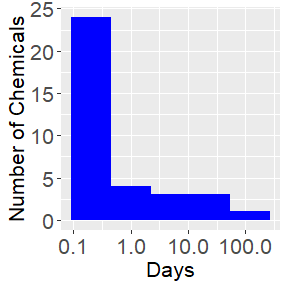## Average vs. maximum concentration

We can compare the average and maximum concentrations at steady state using the average and maximum concentration at steady state vectors, avg and max, from the previous example. The vectors are bound into a data frame and plotted with a line through the origin with a slope of 1 (Pearce et al. (2017) Figure 4).

avg.vs.max <- ggplot(css.data, aes(avg, max)) +
geom_point() + geom_abline() +
scale_x_log10() + scale_y_log10() +
xlab(bquote("Avg. Conc. at Steady State ("*mu*"M)")) +
ylab(bquote("Max. Conc. at Steady State ("*mu*"M)")) +
theme(axis.text = element_text(size = 16),
axis.title = element_text(size = 16))
print(avg.vs.max)